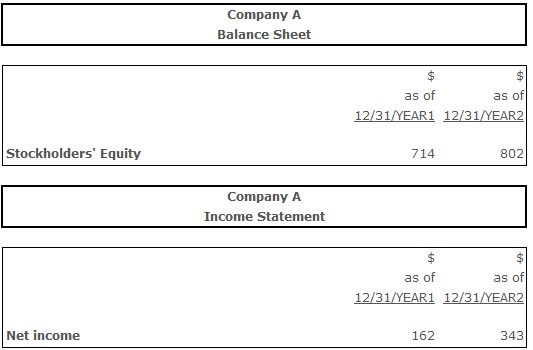Return on Equity

Return on Equity (ROE) – a profitability ratio measuring the ability of a company to generate profits from the investments of the shareholders. The computation formula is flexible enough, and users, who want to measure the return on common equity only may subtract the preferred stock from calculation. This would allow holders of the company's common stock to estimate the return generated by their shares. Calculating the ratio for different periods helps to understand the changes in profitability.

Obviously, higher ratios preferable, because they demonstrate the relative increase of net income, generated by the same amount of equity. Stable rising trend of the return on equity ratio means the increase in company's profit generation ability. However, the decrease in shareholders' equity (which may be caused, for instance, by share buybacks or write-downs) leads to the increase of the return on equity ratio. High level of debt also causes ROE increase, since this means that the company uses credit capital instead of equity as the source of finance. This could be misleading, so for the proper estimation of company's performance debt ratio analysis should be performed in addition to ROE calculation. If debt ratios are normal and ROE demonstrates stable growth - this is a sign of profitable and efficient performance.

Formula(s):

Return on Equity = Net Income Before Noncontrolling Interest and Nonrecurring Items ÷ Total Equity

Return on Equity = Net Profit After Taxes ÷ Equity

Example:Return on Equity (Year 1) = 162 ÷ 714 = 0,22

Return on Equity (Year 2) = 343 ÷ 802 = 0,42

Notable growth of the return on equity ratio from 0,22 in year 1 to 0,42 in year 2 indicates the increase in the value of the stockholders' wealth. This means that the ability of a company to generate the net income from the stockholders’ investments has grown by almost 2 times.

Conclusion:

Return on equity is an important tool, measuring firm's profitability from the stockholders' perspective. Stable growth of the ratio most likely means the increase in company's profitability, when ratio values below 1 would mean unprofitable use of shareholders' equity.